$$\newcommand{\id}{\mathrm{id}}$$ $$\newcommand{\Span}{\mathrm{span}}$$ $$\newcommand{\kernel}{\mathrm{null}\,}$$ $$\newcommand{\range}{\mathrm{range}\,}$$ $$\newcommand{\RealPart}{\mathrm{Re}}$$ $$\newcommand{\ImaginaryPart}{\mathrm{Im}}$$ $$\newcommand{\Argument}{\mathrm{Arg}}$$ $$\newcommand{\norm}{\| #1 \|}$$ $$\newcommand{\inner}{\langle #1, #2 \rangle}$$ $$\newcommand{\Span}{\mathrm{span}}$$

# 9.2.2E: Hyperbolas (Exercises)

$$\newcommand{\vecs}{\overset { \rightharpoonup} {\mathbf{#1}} }$$ $$\newcommand{\vecd}{\overset{-\!-\!\rightharpoonup}{\vphantom{a}\smash {#1}}}$$$$\newcommand{\id}{\mathrm{id}}$$ $$\newcommand{\Span}{\mathrm{span}}$$ $$\newcommand{\kernel}{\mathrm{null}\,}$$ $$\newcommand{\range}{\mathrm{range}\,}$$ $$\newcommand{\RealPart}{\mathrm{Re}}$$ $$\newcommand{\ImaginaryPart}{\mathrm{Im}}$$ $$\newcommand{\Argument}{\mathrm{Arg}}$$ $$\newcommand{\norm}{\| #1 \|}$$ $$\newcommand{\inner}{\langle #1, #2 \rangle}$$ $$\newcommand{\Span}{\mathrm{span}}$$ $$\newcommand{\id}{\mathrm{id}}$$ $$\newcommand{\Span}{\mathrm{span}}$$ $$\newcommand{\kernel}{\mathrm{null}\,}$$ $$\newcommand{\range}{\mathrm{range}\,}$$ $$\newcommand{\RealPart}{\mathrm{Re}}$$ $$\newcommand{\ImaginaryPart}{\mathrm{Im}}$$ $$\newcommand{\Argument}{\mathrm{Arg}}$$ $$\newcommand{\norm}{\| #1 \|}$$ $$\newcommand{\inner}{\langle #1, #2 \rangle}$$ $$\newcommand{\Span}{\mathrm{span}}$$

section 9.2 exercises

Section 9.2 Exercises

In problems 1–4, match each graph to equations A–D.

A. $$\dfrac{x^2}{4} - \dfrac{y^2}{9} = 1$$

B. $$\dfrac{x^2}{9} - \dfrac{y^2}{4} = 1$$

C. $$y^2 - \dfrac{x^2}{9} = 1$$

D. $$\dfrac{y^2}{9} - x^2 = 1$$

1.2.3.4.In problems 5–14, find the vertices, length of the transverse axis, and equations of the asymptotes. Sketch the graph. Check using a graphing utility.

5. $$\dfrac{x^2}{4} - \dfrac{y^2}{25} = 1$$

6. $$\dfrac{y^2}{16} - \dfrac{x^2}{9} = 1$$

7. $$y^2 - \dfrac{x^2}{4} = 1$$

8. $$x^2 - \dfrac{y^2}{25} = 1$$

9. $$x^2 - 9y^2 = 9$$

10. $$y^2 - 4x^2 = 4$$

11. $$9y^2 - 16x^2 = 144$$

12. $$16x^2 - 25y^2 = 400$$

13. $$9x^2 - y^2 = 18$$

14. $$4y^2 - x^2 = 12$$

In problems 15–16, write an equation for the graph.

15.16.In problems 17–22, find the standard form of the equation for a hyperbola satisfying the given conditions.

17. Vertices at (0, 4) and (0, -4); asymptote $$y = \dfrac{1}{2}x$$

18. Vertices at (-6, 0) and (6, 0); asymptote $$y = 3x$$

19. Vertices at (-3, 0) and (3, 0); passes through (5, 8)

20. Vertices at (0, 4) and (0, -4); passes through (6, 5)

21. Asymptote $$y = x$$; passes through (5, 3)

22. Asymptote $$y = x$$; passes through (12, 13)

In problems 23–30, match each graph to equations A–H.

A. $$\dfrac{\left( x - 1 \right)^2}{9} - \dfrac{\left( y - 2 \right)^2}{4} = 1$$

B. $$\dfrac{\left( x + 1 \right)^2}{9} - \dfrac{\left( y + 2 \right)^2}{4} = 1$$

C. $$\dfrac{\left( x + 1 \right)^2}{9} - \dfrac{\left( y + 2 \right)^2}{16} = 1$$

D. $$\dfrac{\left( x - 1 \right)^2}{9} - \dfrac{\left( y - 2 \right)^2}{16} = 1$$

E. $$\dfrac{\left( y - 2 \right)^2}{4} - \dfrac{\left( x - 1 \right)^2}{9} = 1$$

F. $$\dfrac{\left( y + 2 \right)^2}{4} - \dfrac{\left( x + 1 \right)^2}{9} = 1$$

G. $$\dfrac{\left( y + 2 \right)^2}{4} - \dfrac{\left( x + 1 \right)^2}{16} = 1$$

H. $$\dfrac{\left( y - 2 \right)^2}{4} - \dfrac{\left( x - 1 \right)^2}{16} = 1$$

23.24.25.26.27.28.29.30.In problems 31–40, find the center, vertices, length of the transverse axis, and equations of the asymptotes. Sketch the graph. Check using a graphing utility.

31. $$\dfrac{\left( x - 1 \right)^2}{25} - \dfrac{\left( y + 2 \right)^2}{4} = 1$$

32. $$\dfrac{\left( y - 3 \right)^2}{16} - \dfrac{\left( x + 5 \right)^2}{36} = 1$$

33. $$\dfrac{\left( y - 1 \right)^2}{9} - \left( x + 2 \right)^2 = 1$$

34. $$\dfrac{\left( x - 1 \right)^2}{25} - \left( y - 6 \right)^2 = 1$$

35. $$4x^2 - 8x - y^2 = 12$$

36. $$4y^2 + 16y - 9x^2 = 20$$

37. $$4y^2 - 16y - x^2 - 2x = 1$$

38. $$4x^2 - 16x - y^2 + 6y = 29$$

39. $$9x^2 + 36x - 4y^2 + 8y = 4$$

40. $$9y^2 + 36y - 16x^2 - 96x = - 36$$

In problems 41–42, write an equation for the graph.

41.42.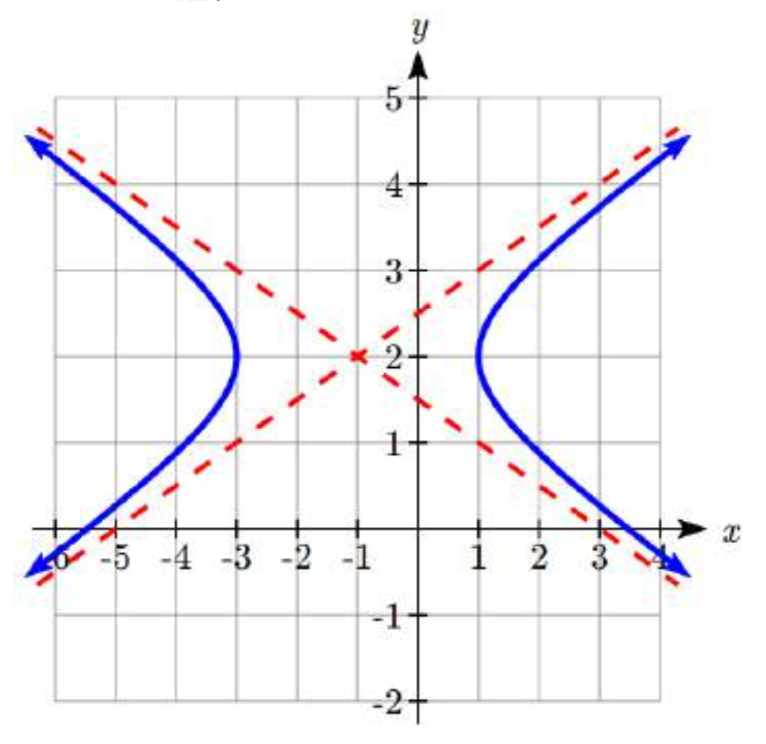In problems 43–44, find the standard form of the equation for a hyperbola satisfying the given conditions.

43. Vertices (-1,-2) and (-1,6); asymptote $$y - 2 = 2\left( {x + 1} \right)$$

44. Vertices (-3,-3) and (5,-3); asymptote $$\;y + 3 = \dfrac{1}{2}\left( {x - 1} \right)$$

In problems 45–48, find the center, vertices, length of the transverse axis, and equations of the asymptotes. Sketch the graph. Check using a graphing utility.

45. $$y = \pm 4\sqrt {9x^2 - 1}$$

46. $$y = \pm \dfrac{1}{4}\sqrt {9x^2 + 1}$$

47. $$y = 1 \pm \dfrac{1}{2}\sqrt {9x^2 + 18x + 10}$$

48. $$= - 1 \pm 2\sqrt {9x^2 - 18x + 8}$$

In problems 49–54, find the foci.

49. $$\dfrac{y^2}{6} - \dfrac{x^2}{19} = 1$$

50. $$x^2 - \dfrac{y^2}{35} = 1$$

51. $$\dfrac{\left( x - 1 \right)^2}{15} - \left( y - 6 \right)^2 = 1$$

52. $$\dfrac{\left( y - 3 \right)^2}{47} - \dfrac{\left( x + 5 \right)^2}{2} = 1$$

53. $$y = 1 \pm \dfrac{4}{3}\sqrt {x^2 + 8x + 25}$$

54. $$y = - 3 \pm \dfrac{12}{5}\sqrt {x^2 - 4x - 21}$$

In problems 55–66, find the standard form of the equation for a hyperbola satisfying the given conditions.

55. Foci (5, 0) and (-5, 0), vertices (4, 0) and (4, 0)

56. Foci (0, 26) and (0, -26), vertices (0, 10) and (0, -10)

57. Focus (0, 13), vertex (0, 12), center (0, 0)

58. Focus (15, 0), vertex (12, 0), center (0, 0)

59. Focus (17, 0) and (-17, 0), asymptotes $$y = \dfrac{8}{15}x$$ and $$y = - \dfrac{8}{15}x$$

60. Focus (0, 25) and (0, 25), asymptotes $$y = \dfrac{24}{7}x$$ and $$y = - \dfrac{24}{7}x$$

61. Focus (10, 0) and (-10, 0), transverse axis length 16

62. Focus (0, 34) and (0, -34), transverse axis length 32

63. Foci (1, 7) and (1, -3), vertices (1, 6) and (1,-2)

64. Foci (4, -2) and (-6, -2), vertices (2, -2) and (-4, -2)

65. Focus (12, 3), vertex (4, 3), center (-1, 3)

66. Focus (-3, 15), vertex (-3, 13), center (-3, -2)

67. LORAN Stations A and B are 100 kilometers apart and send a simultaneous radio signal to a ship. The signal from A arrives 0.0002 seconds before the signal from B. If the signal travels 300,000 kilometers per second, find an equation of the hyperbola on which the ship is positioned if the foci are located at A and B.

68. Thunder and Lightning Anita and Samir are standing 3050 feet apart when they see a bolt of light strike the ground. Anita hears the thunder 0.5 seconds before Samir does. Sound travels at 1100 feet per second. Find an equation of the hyperbola on which the lighting strike is positioned if Anita and Samir are located at the foci.

69. Cooling Tower The cooling tower for a power plant has sides in the shape of a hyperbola. The tower stands 179.6 meters tall. The diameter at the top is 72 meters. At their closest, the sides of the tower are 60 meters apart. Find an equation that models the sides of the cooling tower.70. Calibration A seismologist positions two recording devices 340 feet apart at points A and B. To check the calibration, an explosive is detonated between the devices 90 feet from point A. The time the explosions register on the devices is noted and the difference calculated. A second explosion will be detonated east of point A. How far east should the second explosion be positioned so that the measured time difference is the same as for the first explosion?

71. Target Practice A gun at point A and a target at point B are 200 feet apart. A person at point C hears the gun fire and hit the target at exactly the same time. Find an equation of the hyperbola on which the person is standing if the foci are located at A and B. A fired bullet has a velocity of 2000 feet per second. The speed of sound is 1100 feet per second.

72. Comet Trajectories A comet passes through the solar system following a hyperbolic trajectory with the sun as a focus. The closest it gets to the sun is 3×108 miles. The figure shows the trajectory of the comet, whose path of entry is at a right angle to its path of departure. Find an equation for the comet’s trajectory. Round to two decimal places

.73. The conjugate of the hyperbola $$\dfrac{x^2}{a^2} - \dfrac{y^2}{b^2} = 1$$ is $$\dfrac{x^2}{a^2} - \dfrac{y^2}{b^2} = - 1$$. Show that $$5y^2 - x^2 + 25 = 0$$ is the conjugate of $$x^2 - 5y^2 + 25 = 0$$.

74. The eccentricity $$e$$ of a hyperbola is the ratio $$\dfrac{c}{a}$$, where $$c$$ is the distance of a focus from the center and $$a$$ is the distance of a vertex from the center. Find the eccentricity of $$\dfrac{x^2}{9} - \dfrac{y^2}{16} = 1$$.

75. An equilateral hyperbola is one for which a = b. Find the eccentricity of an equilateral hyperbola.

76. The latus rectum of a hyperbola is a line segment with endpoints on the hyperbola that passes through a focus and is perpendicular to the transverse axis. Show that $$\dfrac{2b^2}{a}$$is the length of the latus rectum of $$\dfrac{x^2}{a^2} - \dfrac{y^2}{b^2} = 1$$.

77. Confocal hyperbolas have the same foci. Show that, for $$0 < k < 6$$, all hyperbolas of the form $$\dfrac{x^2}{k} - \dfrac{y^2}{6 - k} = 1$$ are confocal.

1. B

3. D

5. Vertices $$(\pm 2, 0)$$, transverse length = 4, asymptotes $$y = \pm 5/2x$$,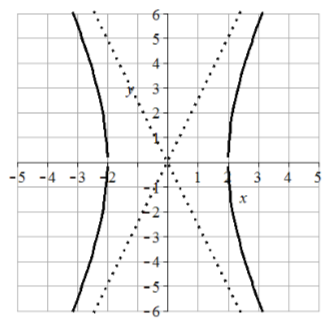7. Vertices $$(0, \pm 1)$$, transverse length = 2, asymptotes $$y = \pm 1/2x$$,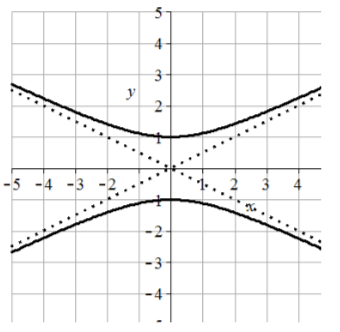9. Vertices $$(\pm 3,0)$$ transverse length = 6, asymptotes $$y =\pm 1/3x$$,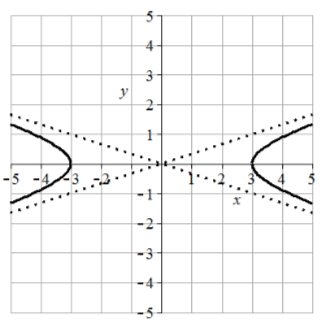11. Vertices $$(0, \pm 4)$$, transverse length = 8, asymptotes $$y = \pm 4/3x$$13. Vertices $$(\pm \sqrt{2}, 0)$$, transverse length = $$2\sqrt{2}$$, asymptotes $$y = \pm 3x$$,15. $$\dfrac{y^2}{4} - \dfrac{x^2}{9} = 1$$

17. $$\dfrac{y^2}{16} - \dfrac{x^2}{64} = 1$$

19. $$\dfrac{y^2}{9} - \dfrac{x^2}{36} = 1$$

21. $$\dfrac{y^2}{16} - \dfrac{x^2}{16} = 1$$

23. C

25. H

27. B

29. A

31. Center (1, -2), vertices (6, -2) and (-4, -2), transverse length = 10, asymptotes $$y = \pm 2/5(x - 1) - 2$$33. Center (-2, 1), vertices (-2, 4) and (-2, -2), transverse length = 6, asymptotes $$y = \pm 3(x + 2) + 1$$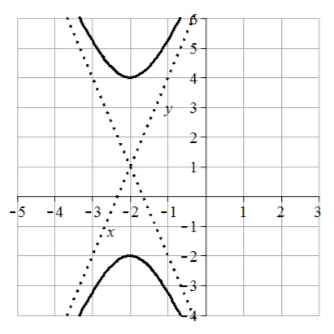35. Center (1, 0), vertices (3, 0) and (-1, 0), transverse length = 4, asymptotes $$y =\pm 2(x-1)$$37. Center (-1, 2), vertices (-1, 4) and (-1, 0), transverse length = 4, asymptotes $$y = \pm 1/2(x + 1) + 2$$39. Center (-2, 1), vertices (0, 1) and (-4, 1), transverse length = 4, asymptotes $$y = \pm 3/2(x + 2) + 1$$41. $$\dfrac{(y + 1)^2}{9} - \dfrac{(x - 4)^2}{4} = 1$$

43. $$\dfrac{(y - 2)^2}{16} - \dfrac{(x + 1)^2}{4} = 1$$

45. Center (0,0), vertices $$(\pm 1/3,0)$$, transverse length = 2/3, asymptotes $$y = \pm 12 x$$46. Center (-1, 1), vertices (-1, 3/2) and (-1, 1/2), transverse length = 1, asymptotes $$y = \pm 3/2 (x + 1) + 1$$49. Foci $$(0, \pm 5)$$

51. Foci (5, 6) and (-3, 6)

53. Foci (-4, 6) and (-4, -4)

55. $$\dfrac{x^2}{16} - \dfrac{y^2}{9} = 1$$

57. $$\dfrac{y^2}{144} - \dfrac{x^2}{25} = 1$$

59. $$\dfrac{x^2}{225} - \dfrac{y^2}{64} = 1$$

61. $$\dfrac{x^2}{64} - \dfrac{y^2}{36} = 1$$

63. $$\dfrac{(y - 2)^2}{16} - \dfrac{(x - 1)^2}{9} = 1$$

65. $$\dfrac{(x + 1)^2}{25} - \dfrac{(y - 3)^2}{144} = 1$$

67. $$\dfrac{x^2}{900} - \dfrac{y^2}{1600} = 1$$

69. $$\dfrac{x^2}{900} - \dfrac{y^2}{14400.3636} = 1$$

71. $$\dfrac{x^2}{3025} - \dfrac{y^2}{6975} = 1$$

73. $$5y^2 - x^2 + 25 = 0$$ can be put in the form $$\dfrac{y^2}{5} - \dfrac{x^2}{25} = -1$$. $$x^2 - 5y^2 + 25 = 0$$ can be put in the form $$\dfrac{y^2}{5} - \dfrac{x^2}{25} = 1$$ showing they are conjugate.

75. $$\sqrt{2}$$

77. No matter the value of $$k$$, the foci are at $$(\pm \sqrt{6}, 0)$$